# Monotonically normal space

(diff) ← Older revision | Latest revision (diff) | Newer revision → (diff)
This article defines a property of topological spaces: a property that can be evaluated to true/false for any topological space|View a complete list of properties of topological spaces
This is a variation of normality. View other variations of normality

## Definition

### Definition with symbols

A topological space$X$ is termed monotonically normal if there exists an operator$G$ from ordered pairs of disjoint closed sets to open sets, such that:

• For any disjoint closed subsets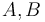$A,B$,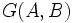$G(A,B)$ contains$A$ and its closure is disjoint from$B$
• If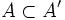$A \subset A'$ and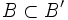$B \subset B'$ with all four sets being closed, and$B$ disjoint from$B'$, we have: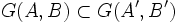$G(A,B) \subset G(A',B')$

This is the monotonicity condition. Such an operator$G$ is termed a monotone normality operator.

## Metaproperties

### Hereditariness

This property of topological spaces is hereditary, or subspace-closed. In other words, any subspace (subset with the subspace topology) of a topological space with this property also has this property.
View other subspace-hereditary properties of topological spaces

Any subspace of a monotonically normal space is monotonically normal.